## UPPCL AE 2022

 Question 1
Which of the following sequences of operations is followed in the instruction cycle?
 A 00: Fetch Cycle 00: Execute Cycle 10: Interrupt Cycle 11: Indirect Cycle B 00: Fetch Cycle 01: Interrupt Cycle 10: Indirect Cycle 11: Execute Cycle C 00: Fetch Cycle 01: Execute Cycle 10: Indirect Cycle 11: Interrupt Cycle D 00: Fetch Cycle 01: Indirect Cycle 10: Execute Cycle 11: Interrupt Cycle
UPPCL AE 2022
 Question 2
Consider the Grammar G (V-(S, A,B,C), T-(a,b),S,P) where V is a non-empty set of variables or non-terminals, T is a set of terminals, S is a start symbol and P is a set of production des given as follows. Which of the following strings is in L(G)
 A aaa B ababbab C null string D bbb
 Question 3
Let F(x,y) denote the predicate ‘ y is a friend of x’. Which of the following correctly describes' It is impossible that someone does not have any friends’?
 A For all y there exists x (F(x, y)) B There exists y for all x (F(x, y)) C There exists x for all y (F(x, y)) D For all x there exists y (F(x, y))
 Question 4
2 doctors and 10 nurses attend a small conference. All 12 names are put in a hat and 4 names are randomly picked without replacement. The probability that 1 doctor and 3 nurses are picked is:
 ABCDQuestion 5
In a __________ graph, vertices can be partitioned into two subsets V1 and V2 suchthat no edge has both endpoints in the same subset, and every possible edge that could connect vertices in different subsets is part of the graph.
 A complete bipartite B clique C complete D bipartite
 Question 6
Let Grammar G(V=(5).T (ab)SP)stere V is a no-empty set of variables or non-terminals. T is a set of terminals. S is a start symbol. λ is a null string and is a set of production rules. If na (w), (w) nb represents the number of a's and b'a in string w, then the language derived from set of production rules
 A {W ∈ {a,b}* : na (w) = nb(w)} B {W ∈ {a, b}* : na (w) # nb (w)} C {W ∈ {a, b}* : na (w) < nb(w)} D {W ∈ {a, b}* : na (w) > nb(w)}
 Question 7
Literals can also be called
 A constants B keywords C identifiers D special characters
 Question 8
Simplify the logic expression F = a 'bc + a 'bc' + ac.
 A b + ca' = 1 B ab + b 'c=1 C a + b' c D a 'b + ac
 Question 9
Consider the following schema (staff, name, street, city) serves(name, c_ name, salary) company (c_ name, city) manages (name, manager-name) Find the names and cities of the residence of all the staffs who work for Shankar.
 ABC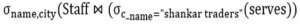DQuestion 10
Which of the following is the application of stack data structure?
 A data transfer B resource scheduling C disk scheduling D evaluation of expressions
 Question 11
Which of the following principles is used by divide and conquer technique?
 A Recursively define the values of optimal solutions B Divide the problem into a number of subproblems C An equation or inequality describes a function in terms of its values on smaller inputs D Construct an optimal solution from computed information
 Question 12
The number of elements in the adjacency matrix of a graph having 6 vertices is_______
 A 36 B 12 C 216 D 24
 Question 13
Find the combination of the inputs (X and Y) for which the Q output is set to 1 for the latch as shown.
 A 1 ,0 B 1, 1 C 0, 1 D 0,0
 Question 14
Which of the following is a partition of the set S = {2,4,6,8,10}?
 A {2, 4}, {2, 6, 8}, {10} B {4, 6}, {4, 8, 10} C {2, 8}, {4}, {6, 10} D {2, 10}, {4}, (6}
 Question 15
For which of the following functions is Rolle’s theorem applicable?A f(x)=x3 in [1,2] B f(x)=x2 in [-1,1] C f(x) = tan x in [0, π] D f(x)=x13 in [−1,1] 1/3
 Question 16
 A Memory Buffer Register (MBR) B Address register C Memory Address Register (MAR) D Program Counter (PC)
 Question 17
In which of the following gates, the output is 0 if and only if at least one input is 0?
 A NOR B OR C XOR D AND
 Question 18
Which of the following is NOT a property of context free language that can be generated from context free grammar G ?
 A There are no productions of the form ABC where A and B are variables B Each variable and each terminal of G appears in the derivation of some word in L. C There are no productions of the form A-→> B where A and B are variables D If is not in L, there needs be no productions of the form A- £.
 Question 19
In dynamic programming, the technique of storing the previously calculated values in called:
 A storing value property B mapping dynamic programming paradigm C saving value property D Memorization
 Question 20
For all sets A, B, C, which of the following does NOT hold?
 A (B ∩ C) ∪ A = (B ∪ A) ∩ (C ∪ A) B A ∩ B = B ∩ A C A ∪ (B ∪ C) = (A ∪ B) ∪ C D A ∩ (B ∩ C) = (A ∩ B) ∪ C
 Question 21
_______are very versatile and are a basic component of inter process and intersystem communication. They also provide point-to-point, two-way communication between two processes.
 A Monitors B Shared memories C Sockets D Semaphores
 Question 22
The number 101010101010 is a 12-bit binary number in 2’s complement form. If it is stored in a 16-bit register, with what would you fill bit 12 to bit 15 (4-bits), so that the value of the number is unchanged?
 A 0101 B 0000 C 1111 D 1010
 Question 23
The giver table describes the rate of economic growth (x) and the rate of return on the S&P 500(y) of a sample. The covariance between these two is :A 1.36 B 1.53 C 1.47 D 1.27
 Question 24
Which is the next step that comes after the intermediate code generator in the phases of a compiler?
 A Machine - independent code optimizer B Semantic analyzer C Machine - dependent code optimizer D Code generator
 Question 25
Match the following pairs with respect to 10 G Ethernet: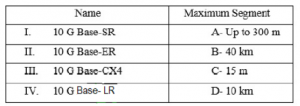A I - A, II - B, III - D, IV - C B I - A, II - D, III - B, IV - C C I - A, II - B, III - C, IV - D D I - A, II - C, III - B, IV - D
 Question 26
The identity elements of OR and AND operations are ______ and ______, respectively.
 A zero, zero B one, zero C one, one D zero, one
 Question 27
The dual of the expression x + x2 = 1 is:
 A x - x2 = 1 B x. x2 = 0 C x. x1 = 1 D x - x = 0
 Question 28
Which of the following is a scalar matrix? (given k is constant)
 AB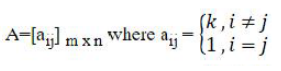CDQuestion 29
Which of the following is a decidable problem?
 A Determine whether a language generated by Turing Machine M is finite. B Determine whether a language L generated by Unrestricted Grammar is empty. C Determine whether language over ∑ (where ∑ is a set of input alphabet) is not recursively enumerable. D Determine whether a context sensitive grammar accepts the input string
 Question 30
The time complexity of constructing a single-tape Turing Machine and a two-tape Turing Machine for the language L = {a" b": n ≥ 1), respectively, are:
 A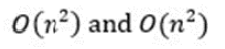BCDQuestion 31
Protocols in which the sender sends one frame and then waits for an acknowledgement before proceeding are called protocols.
 A stop-and-wait B Go-back-n C store and forward D sliding window
 Question 32
A complete graph G with 5 vertices has___________ spanning trees. Ans:
 A 125 B 15 C 3 D 25
 Question 33
Consider the grammar G (V = {S, A, B, C, D, E, T = { a, b }, S, p ) where V is a non -empty set of variables or non- terminals, T is a set of terminals, S is a start symbol and P is a set of production rules given as follows:
S→AB,A →a, B→C, B →b, C →D,D,→E,E →a.
The equivalent grammar after eliminating the unit productions is:
 A S→AB, A →a, B→C, B →b, C →a, D ,→E, E →a B S→AB, A → a, B →a, B →b, C →a, D →a, E → a C S→BC, A →a, B → a, Bb, C →a, D → E, E → a D S →ab, A →a, B →a, B → b, Ca, D →E, E → a.
 Question 34
Suppose a d-regular graph on n vertices (n is even) is disconnected. Which of the following can be the maximum value of d?
 AB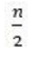C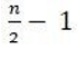DQuestion 35
The type of information stored in computer words______________
 A is data only B are data and instruction C depends on the type of memory D is instruction only
 Question 36
Which of the following is a process that reduces computing time but increases the amount of memory needed?
 A Lookup tables or recalculation B Compressed data C Re-rendering D Smaller code
 Question 37
A string of terminals in the context-free grammar is represented by:
 A a combination of lowercase Greek and uppercase letters B uppercase letters C lowercase Greek letters D lowercase letters
 Question 38
Find the minimum number of additions and multiplications needed to evaluate a degree 5 polynomial at any point x0.
 Question 39
Using a dual 8:1 MUX, what are the extra logic gates required to implement a full- adder?
 A One 2-input XOR B One 2-input AND C One 2-input OR D None
 Question 40
The linear transformation has a matrix.if it transforms all the points of circle with equation x2 + Y2 =4,then the curve is:
 A 9x2 + 4y2 =288 B 9x2 + 4y2 = 24 C x2 + y2 = 12 D 9x2 + 4y2 = 576
 Question 41A 12 B 11 C 10 D 13
 Question 42
Each field of k bits allows for________________ micro-operations. Ans
 A k B 2k+1 C 2k – 1 D 2k
 Question 43
The task of the instruction: Load the content of memory location MI to register RI. There are five control steps after fetch) that are required to execute the instruction "LOAD RI, (M)", as given below.
2 WAFC
3._______
4. WMFC
5. MDRout, Rin
Which of the following fits appropriately in step 3 of the given set of instructions?
 A IRout, MARin, Read B MDRin, MARin C MDRout, MARin D MDRout, MARout
 Question 44
UNION operator results in which of the following?
 A Taking distinct data from the relations. B Taking data that is not common from the relations. C Taking all data from the relations. D Taking common data from the relations.
 Question 45
Which of the following options is true in the case of a two-bus organization?
 A In a two-bus organization, there are two buses. The general-purpose register can read/write from both the buses. In this case, two operands can be fetched at the same time because of the two buses – one bus fetch operand for ALU and another bus fetch for register B In a two-bus organization, there are two buses. The general-purpose register can only write from both the buses. In this case, two operands can be fetched at the same time because of the two buses – one bus fetch operand for ALU and another bus fetch for register. C In a two-bus organization, there are two buses. The general-purpose register can only read from both the buses. In this case, two operands can be fetched at the same time because of the two buses – one bus fetch operand for ALU and another bus fetch for register. D In a two-bus organization, there are two buses. The Th general-purpose register can only write from both the buses. In this case, two operands can be fetched at the same time because of the two buses – one bus fetch operand for ALU and another bus fetch for memory.
 Question 46A Language generated from production P₁ of Grammar is infinite, but the language generated from production P₂ is always finite. B Language generated from production P₁ of Grammar is finite, but the language generated from production Grammar is infinite C Language generated from productions P₁ and P₂ are always infinite D KAMR Language generated from productions P₁ and P₂are always finite.
 Question 47
A binary search tree which provides the smallest possible search time for a given sequence of accesses is:
 A Optimal Binary Search Tree B Self-Balancing Binary Search Tree C Balanced Binary Tree D AVL tree
 Question 48
ADD R1,R2 instruction is an example of which of the following addressing modes?
 Question 49
If 2a + 3b +6c=0, then ax2+bx+c = 0 has at least one root in:
 A (1 ,2 ) B (0 ,1) C (2 ,3) D (-1, 0)
 Question 50
What is the space complexity of the following piece of code?
for ( int i=0;i< N;i++)
V. push _ back(i);
 A O(loan) B O(n) C O(1) D O(nlogg)
There are 50 questions to complete.

Register Now